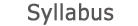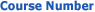0509-2846 Partial Differential Equations2019 - 2020View groups
Course description
Credit Points: 2.5
Prerequisites: Ordinary Differential Equations; Complex Functions; Harmonic
Analysis.
The forced string equation. Initial conditions and boundary conditions (free, forces, and fixed end), derived from physical principles. Uniqueness. The d'Alembert solution for infinite string. Characteristic lines. Reflections from free and fixed ends. Stability. The well-posedness of the string problem. Solutions by separation of variables. Standing waves. Impulse response. The Sturm-Liouville problem. Second order equations in two independent variables and their classification. Canonical forms. The Laplace and Poisson equations in classical continuum theory. Green theorem. Uniqueness for boundary value problems. The maximum principle. The Cauchy problem. Ill-posed Cauchy problem. Uniqueness theorem for the heat equation. Seperation of variables for the heat equation. Laplace equation in a disk and in rectangular domains. Green function for Sturm-Liouville equation. Completeness of the eigenfunctions. Green function for the Dirichlet problem in the Poisson equation. Multi-dimensional problems and their solutions using separation of variables, multi-dimensional Fourier series, and integral transforms. Specific examples: circular membrane.

accessibility declaration